# Mass + ratio - math problems

#### Number of problems found: 45

• Brass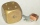Brass is an alloy of copper and zinc in a ratio of 3: 2. How many grams does a component that required 270 g of copper weigh?
• Density - simple exampleMaterial of density of 1485 kg/m3 occupies a container volume of 65 cm3. What is its mass?
• Carbon dioxide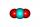Calculate how many grams of oxygen are in 50 g of carbon dioxide CO2. Relative atomic mass of oxygen is 16 and of carbon is 12.
• Vegetable meal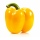The cook was doing meal - in ratio 4: 3: 1 mix tomatoes: pepper: onion. Onions were 5 kg less than peppers. How many kgs of tomatoes did he need to prepare the meal?
• Ratio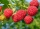Alena collected 7.8 kg of blueberries, 2.6 kg of blackberries, and 3.9 kg of cranberries. Express the ratio in the smallest natural numbers in this order.
• CoinsThe money - coins are minted from the hardest bronze, which contains copper and tin in a ratio of 41: 9. How much copper and tin are in 2kg of bronze money?
• Mixture of nuts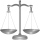Alice bought a mixture of nuts containing cashew nuts, hazelnuts and peanuts in a ratio of 1: 2: 3. Calculate the weight in grams of the whole mixture if the peanuts weigh 90 g.
• Tea blendTea blends are maked from two kinds of tea. In standard tea mixture are two teas in the ratio 1:3 and 40 g costs 42 CZK. In the premium tea mixture are weighing two teas in the ratio 1:1 and 50 grams costs 60 CZK. How much cost 10 grams of more expensive
• SupermarketIn a local supermarket, 3/5 kilograms of squid cost 156.00. How do 4 kilograms of squid cost?
• Nutballs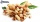The dough for nutballs contains, among other things, two basic raw materials: flour and nuts in a ratio of 2:1. How much flour and how many nuts are needed for 1 kg of dough if "other" is 100g?
• Gas consumption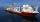The vessel consume 100 tons of gas in 250 miles. How many fuel will the vessel consume if it travels 400 miles?
• Potatoes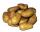Potatoes contain 78.6% starch. How many potatoes need to obtain 27 kg of starch?
• Mixture of nutsThe mixture of nuts should be prepared from almonds, peanuts and cashew nuts ratio 1: 2: 3 (respectively). The price of almonds is 150 CZK/kg, the price of peanuts is 140 CZK/kg and the price of cashew nuts is 180 CZK/kg. The price of the mixture is deter
• WeightsMarry and John together weighing 49 kg. Their weights are in ratio 1:6. Determine their weights.
• Rails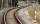18 m railway weighs 1260 kg. How much weighs 100 m of welded railways?
• Cows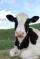20 cows has food for 60 days. We reduce the dose to two-thirds for 18 cows. How many days will be fed?
• Carrot seed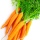Carrot seed germination is 85%, weight 1000 carrots seeds are 2.4g. How many seeds are likely to germinate if we sow 8g of seeds?
• Kilo candyOne kilogram of chocolate candy cost 10 euros. Kate buys 250 grams and had the 8 pack of candy. How much does cost one bonbon?
• The pigletThe weight of the piglet grew regularly by five kilograms for four months. Determine the proportion by weight of the piglet in each month, if the first weighed 35 kg.
• Metal alloy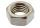What is the ratio of metals in the alloy that is in the 50 tonnes of steel to 30 kg nickel?

Do you have an interesting mathematical word problem that you can't solve it? Submit a math problem, and we can try to solve it.

We will send a solution to your e-mail address. Solved examples are also published here. Please enter the e-mail correctly and check whether you don't have a full mailbox.

Please do not submit problems from current active competitions such as Mathematical Olympiad, correspondence seminars etc...

Check out our ratio calculator. Mass - math word problems. Ratio - math word problems.